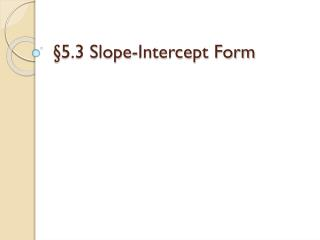DownloadDownload Presentation§5.3 Slope-Intercept Form

# §5.3 Slope-Intercept Form

Télécharger la présentation## §5.3 Slope-Intercept Form

- - - - - - - - - - - - - - - - - - - - - - - - - - - E N D - - - - - - - - - - - - - - - - - - - - - - - - - - -
##### Presentation Transcript

1. §5.3 Slope-Intercept Form

2. Evaluate each expression. 1. 6a + 3 for a = 2 2. –2x – 5 for x = 3 3.x + 2 for x = 16 4. 0.2x + 2 for x = 15 Solve each equation for y. 5.y – 5 = 4x6.y + 2x = 7 7. 2y + 6 = –8x 1 4 Warm-Up

3. Warm-up (solutions) 1 4 1 4 2y y 1. 6a + 3 for a = 2: 6(2) + 3 = 12 + 3 = 15 2. –2x – 5 for x = 3: –2(3) – 5 = –6 – 5 = –11 3.x + 2 for x = 16: (16) + 2 = 4 + 2 = 6 4. 0.2x + 2 for x = 15: 0.2(15) + 2 = 3 + 2 = 5 5.y – 5 = 4x6.y + 2x = 7 y – 5 + 5 = 4x + 5 y + 2x – 2x = 7 – 2x y = 4x + 5 y = –2x + 7 7. 2y + 6 = –8x 2y + 6 – 6 = –8x – 6 2y = –8x – 6 = y = –4x – 3 –8x – 6 2

4. Vocabulary Linear Equation: an equation whose graph forms a straight line. y-Intercept: the y-coordinate of the point where a line crosses the y-axis (vertical axis). Slope-Intercept Form: a linear equation of a non-vertical line written as y = mx + b, where m is the slope and b is the y-intercept.

5. Example 1: Identifying Slope and y-intercept Use the Slope-Intercept form. mx + b y = y = 2x – 3 What are the slope and y-intercept of y = 2x – 3? The slope is 2; the y-intercept is –3.

6. Example 2: Writing an Equation in Slope-Intercept Form 2 5 Use the slope-intercept form. Substitute for m and4 for b. y = mx + b 2 5 2 5 y = x + 4 Write an equation of the line with slope and y-intercept 4.

7. Example 3: Writing an Equation From a Graph Write an equation for the line. Step 1 Find the slope. Two points on the line are (0, 1) and (3, –1). –1 – 1 3– 0 slope = 2 3 = – y = mx + b Step 2 Write an equation in slope– intercept form. The y-intercept is 1. 2 3 y = – x + 1 Substitute – for mand 1 for b. 2 3

8. Example 4: Writing an Equation From Two Points What equation in slope-intercept form represents the line that passes through the points (0, 3) and (5, 2)? Step 1Use the two points to calculate the slope of the line. m = = = y1 – y23 – 21 x1 – x20 – 5 -5 Step 2Use the slope and the coordinates of one of the points to find b. y= mx + b 3 = (-0.2)(0) + b 3 = 0 + b 3 = b Step 3Substitute the slope and y-intercept into the slope-intercept form. y= mx + b y = -0.2x + 3 y = -0.2x + 3

9. Example 5: Graphing a Linear Equation Step 2 The slope is . Use slope to plot a second point. Step 3 Draw a line through the two points. Step 1 The y-intercept is –2. So plot a point at (0,–2). 1 3 1 3 Graph y = x – 2.

10. Assignment: Pg. 312 7-35 Left